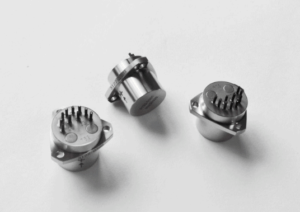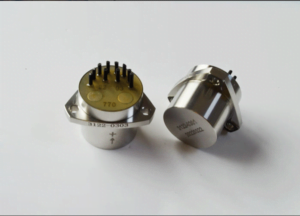Application

# How does the Quartz Accelerometer Work?Accelerometers are used to measure the static acceleration of gravity, so that the Angle of deviation of the object under test from the vertical direction can be determined, as well as the measurement of the dynamic acceleration due to impact, motion, collision or vibration (i.e. low and low frequency vibration of tens of amplitudes).

How does the accelerometer work when measuring vibration? The accelerometer measures directly on the vibrating object, thereby converting the vibrating energy into an electrical signal proportional to the instantaneous acceleration of the object.

Accelerometer action? Vibration measurement is commonly used to diagnose the operating condition of machines, equipment or structures subjected to high stress, such as steel mast structures, Bridges or building structures. Accelerometers are also used to protect hard drives from damage, as well as in medical and sports equipment, cameras and camcorders, smartphones, remote controls, controllers and navigation systems.

What is an accelerometer? It’s nothing more than an acceleration transducer that measures its own motion through space. There are three basic types of accelerometers, which are described in more detail later in this article.

###### How the quartz accelerometer works

The way accelerometers work is not too complicated. It measures acceleration in units of g and can be measured on one, two, or three planes. Currently, the most commonly used is the 3-axis accelerometer, whose structure consists of three accelerometers, each measuring acceleration in a different direction in the X, Y, and Z planes.

If the acceleration in any plane acts in the opposite direction to that which the sensor is pointing, the accelerometer will measure the acceleration in negative values. Otherwise, acceleration is measured as a positive value.

If the accelerometer is not affected by any external acceleration, the device will only measure the acceleration with respect to the Earth, i.e., gravity. Assuming the 3-axis accelerometer is placed in such a way that the sensor on the X-axis points to the left, the sensor on the Y-axis points down, and the sensor on the z-axis points forward and is not affected by any force, the accelerometer will return the following values: X = 0 g, Y = 1 g, and Z = 0 g. If the same accelerometer is deflected to the left, its reading will show: X = 1 g, Y = 0 g, Z = 0 g. Similarly, when tilted to the right, the x-plane will return X = -1 g. The given accelerometer measurement correlation is realized by the system algorithm that monitors the accelerometer’s work.

In inertial navigation system, the measurement of acceleration is realized by the accelerometer. Acceleration is a physical quantity used to reflect the speed change state of an object in the process of movement. It cannot be measured directly. It is usually measured indirectly by something that can be measured directly, such as time or displacement. Current acceleration sensors (accelerometers) use indirect measurement and force balance to obtain the acceleration of an object. The working principle of the accelerometer is based on Newton’s second law of motion: the acceleration of an object is dependent upon two variables-the net force acting upon the object and the mass of the object.

Therefore, the output of the acceleration sensor reflects the net force of the sensitive balance in the sensor in the whole space, mainly including inertial force and gravity. When the object moves in space, the sensor can obtain the acceleration of the object in real-time. The instantaneous velocity of the object can be obtained by integrating the acceleration once, and the relative position of the object can be obtained by integrating the velocity once. Using these three parameters, the motion trajectory of the object can be described.Quartz flexible accelerometer and its development status as a kind of force feedback pendulum accelerometer, quartz flexible accelerometer is developed on the basis of the liquid floating pendulum accelerometer. The main difference between the two is that the detection quality of the quartz flexible accelerometer is not floating, but is elastically connected to the flexible beam support, introducing elastic moment. Therefore, this kind of accelerometer has higher accuracy, strong anti-interference ability Large measuring range and strong overload capacity. The performance of flexible material directly affects the performance of the accelerometer. There are two kinds of materials that can be used as the flexible pendulum: metal and quartz. The traditional liquid floating pendulum accelerometer pendulum uses metal materials, while the thermal expansion coefficient of quartz is much smaller than that of steel, and the material performance is better than that of metal. Moreover, quartz has high anti-fatigue strength and small hysteresis, so it is very suitable to be used as the pendulum of the accelerometer. As soon as the quartz flexible accelerometer came out, it quickly replaced the liquid floating accelerometer and became an indispensable key device in the inertial navigation and guidance system.

At present, the quartz flexible accelerometer has been widely used in the measurement of various linear acceleration and vibration acceleration, as well as the measurement of velocity, distance, angular velocity, angular displacement and other parameters. It has been successfully used in military and civilian fields such as satellite microgravity measurement systems, high-precision inertial navigation systems, rock and soil-based borehole measurement and oil drilling, continuous inclinometer system, carrier rocket, ballistic missile and spacecraft.